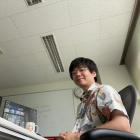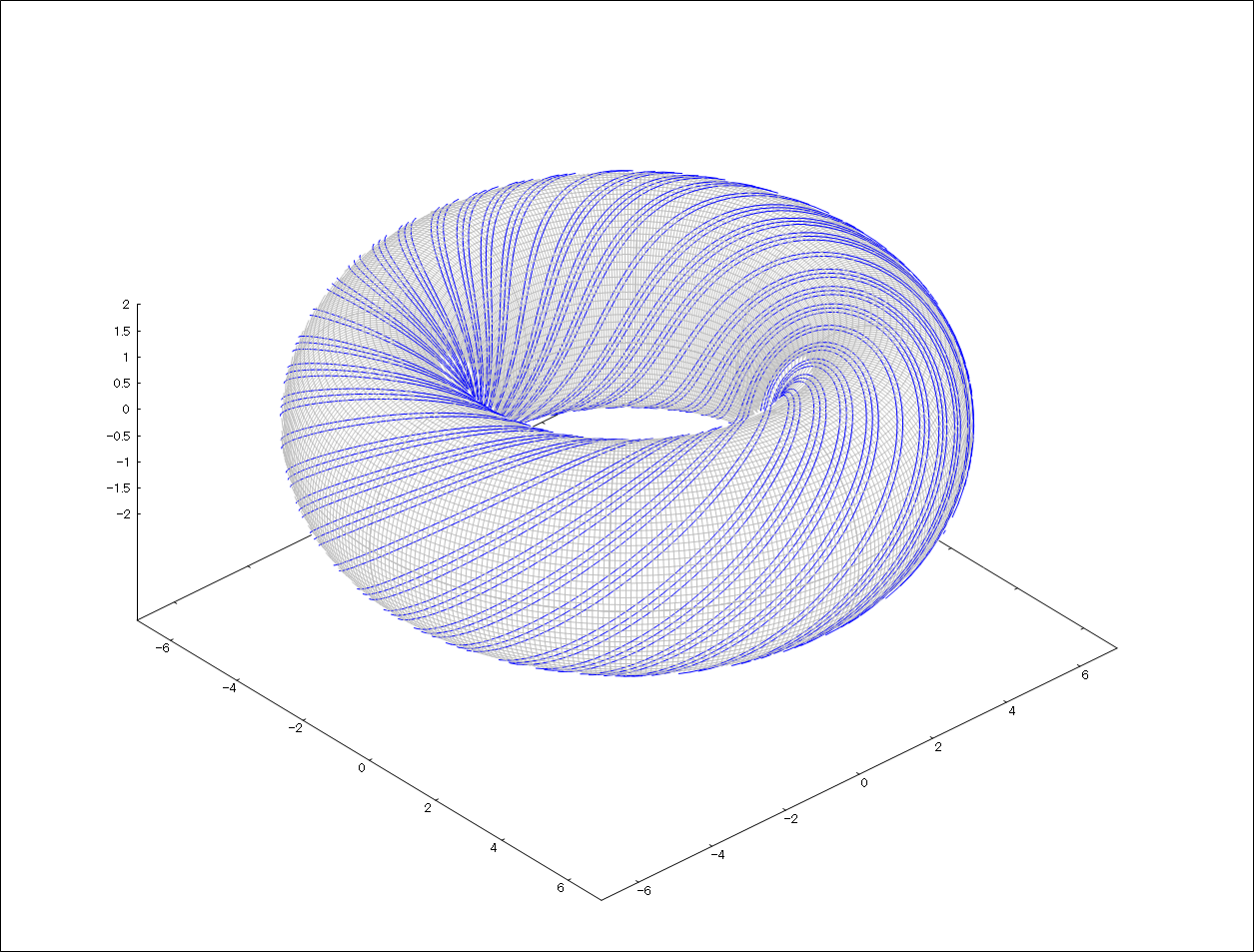Welcome to the Curious World of Science!
Research of Science at Shinshu University.Yosuke KUBOTA

Mathematics

SOAR：View SOAR site

# From noncommutative geometry to everything

## Current research theme: Higher index theory

A motivation of my research is to apply the idea of noncommutative geometry to various area of mathematics. There are several different kind of topics I'm currently working on. Here I'm going to introduce only one of them. It is about the topology ("soft geometry") of manifolds (a kind of space or shape).

About 10 years ago, it was featured in the news as one of the greatest achievement in this century that Perelman proved the Poincaré conjecture. On the other hand, the same conjecture for manifolds of dimension 5 or more has been proved long before that, in 1960s. In general, it is known that the theoretical behavior of the topology of manifolds differs largely in dimension 4 or less and 5 or more. By this reason we often call manifolds of dimension 4 or less "low-dimensional" and of dimension 5 or more "high-dimensional". The research subject which I'm interested in is the topology of high-dimensional manifolds.

There is an important and interesting slogan in high-dimensional topology, which says that "the complexity of the topology of high-dimensional manifolds corresponds to that of its fundamental group". Here "group" is an algebraic object commonly used in mathematics. Shortly, the set of loops in the space under consideration forms a group (after identifying two loops which are topologically the same), which is called the fundamental group.

There is an area of mathematics other than topology, which is called geometric group theory, studying the "shape" and its complexity of the groups. The above slogan says that if the fundamental group is easy in the sense of geometric group theory, the topology of the space under consideration is also easy, and vice versa.

The analysis/geometry of the fundamental group and topology of spaces superficially looks very different. It is really non-trivial that they are deeply related. The theory bridging these two different area is the noncommutative geometry or higher index theory, my main research area.

## Research area: Noncommutative geometry

The origin of noncommutative geometry goes back to the Atiyah-Singer index theorem;

$\mathop{\mathrm{Index}}(D) = (-1)^n \cdot \int_{T^*M} \mathop{\mathrm{ch}}(\sigma(D)) \cdot \hat{A}(M)^2.$

This theorem claims that the dimension of the space of solutions of a kind of differential equation on the space coincides with a topological invariant called (the integral of ) a characteristic class. This beautiful theorem locates at the junction of the analysis and geometry-topology. Atiyah and Singer have developed a magnificent theory called the index theory involving functional analysis and topology. After more than 50 years from their proof, this theory still has been deepened and sophisticated. A remarkable turning point was that the theory of operator algebra is taken in for bridging functional analysis and topology. This makes index theory larger and developed in a wider direction involving differential topology, operator algebra, representation theory and geometric group theory. In particular, it brought an essential progress in the study of the Novikov conjecture, which has been thought to be an important problem in differential topology.

The Noncommutative Geometry is a magnificent program proposed by A. Connes. Here the terminology "noncommutative" just mean that the commutative low $AB=BA$ of product is possibly broken. This is a basic property of matrices arising in linear algebra, and in fact noncommutative geometry mainly studies operators, i.e., infinite size matrices. The concept is to "study a noncommutative (operator) algebra like we study a space". Indeed, it is known that a C*-algebra (a kind of operator algebras) is known to be thought of as a "noncommutative generalization" of a space. Then it is a natural idea to extend techniques for studying spaces to a wider category; C*-algebras. Based on this idea, Connes succeeds to include some dynamical systems like group actions or foliations to the range of geometric studies. Moreover, the noncommutative geometry also aims to describe the "quantum mechanical space" (where noncommutativity of the position and momentum plays an essential role).

A typical example of this "noncommutative space" is the noncommutative torus (or the irrational rotation algebra). The algebra of functions on the $2$-dimensional torus

$\{ (z,w) \in \mathbb{C}^2 \mid |z|=1,|w|=1\}$

is roughly identified with the $2$-variable polynomial algebra $\mathbb{C}[z,z^{-1}, w, w^{-1}]$. Here notice that $z$ and $w$ satisfies the commutative law, i.e., $zw=wz$. We modify this relation as

$zw=e^{i\theta }wz$

to get the noncommutative torus. The noncommutative torus is a universal mathematical object, arising even in the theory of condensed-matter physics (if you are interested in, you can google it by the keyword "Hofstadter's butterfly"). Moreover, in relation to the theory of Connes, it is nothing but the "noncommutative space" corresponding to the dynamical system called the Kronecker foliation (see the figure below).